International
Tables for
Crystallography
Volume H
Powder diffraction
Edited by C. J. Gilmore, J. A. Kaduk and H. Schenk

International Tables for Crystallography (2018). Vol. H, ch. 2.5, pp. 119-123

## Section 2.5.2. Fundamentals

B. B. Hea*

aBruker AXS Inc., 5465 E. Cheryl Parkway, Madison, WI 53711, USA
Correspondence e-mail: bob.he@bruker.com

### 2.5.2. Fundamentals

| top | pdf |

A conventional powder-diffraction pattern is displayed as the scattering intensity versus scattering angle 2θ or d-spacing. A 2D-XRD pattern contains the scattering-intensity distribution as a function of two orthogonal dimensions. One dimension can be expressed in 2θ, which can be interpreted by Bragg's law. The distribution in the dimension orthogonal to 2θ contains additional information, such as the orientation distribution, strain states, and crystallite-size and -shape distribution. In order to understand and analyse 2D diffraction data, new geometry conventions and algorithms are introduced. The geometry conventions and algorithms used for 2D-XRD should also be consistent with conventional XRD, so that many existing concepts and algorithms are still valid when 2D diffraction data are used.

The geometry of a 2D-XRD system can be explained using three distinguishable and interrelated geometry spaces, each defined by a set of parameters (He, 2003). The three geometry spaces are the diffraction space, detector space and sample space. The laboratory coordinate system XL, YL, ZL is the basis of all three spaces. Although the three spaces are interrelated, the definitions and corresponding parameters should not be confused. Except for a few parameters introduced specifically for 2D-XRD, many of these parameters are used in conventional X-ray diffraction systems. Therefore, the same definitions are maintained for consistency. The three-circle goniometer in Eulerian geometry is the most commonly used, and all the algorithms for data interpretation and analysis in this chapter are based on Eulerian geometry. The algorithms can be developed for the geometries of other types (such as kappa) by following the same strategies.

| top | pdf |

#### 2.5.2.1.1. Diffraction cones in laboratory coordinates

| top | pdf |

Fig. 2.5.3(a) describes the geometric definition of diffraction cones in the laboratory coordinate system XL, YL, ZL. The laboratory coordinate system is a Cartesian coordinate system. The plane given by XL and YL is the diffractometer plane. The axis ZL is perpendicular to the diffractometer plane. The axes XL, YL and ZL form a right-handed rectangular coordinate system with the origin at the instrument centre. The incident X-ray beam propagates along the XL axis, which is also the rotation axis of all diffraction cones. The apex angles of the cones are determined by the 2θ values given by the Bragg equation. The apex angles are twice the 2θ values for forward reflection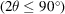and twice the value of 180° − 2θ for backward reflection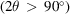. For clarity, only one diffraction cone of forward reflection is displayed. The γ angle is the azimuthal angle from the origin at the six o'clock direction with a right-handed rotation axis along the opposite direction of incident beam (−XL direction). A given γ value defines a half plane with the XL axis as the edge; this will be referred to as the γ plane hereafter. The diffractometer plane consists of two γ planes at γ = 90° and γ = 270°. Therefore many equations developed for 2D-XRD should also apply to conventional XRD if the γ angle is given as a constant of 90° or 270°. A pair of γ and 2θ values represents the direction of a diffracted beam. The γ angle takes a value of 0 to 360° for a complete diffraction ring with a constant 2θ value. The γ and 2θ angles form a spherical coordinate system which covers all the directions from the origin of sample (instrument centre). The γ–2θ system is fixed in the laboratory system XL, YL, ZL, which is independent of the sample orientation and detector position in the goniometer. 2θ and γ are referred to as the diffraction-space parameters. In the laboratory coordinate system XL, YL, ZL, the surface of a diffraction cone can be mathematically expressed as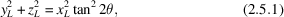with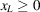or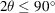for forward-diffraction cones and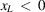or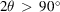for backward-diffraction cones.Figure 2.5.3 | top | pdf |The diffraction cone and the corresponding diffraction-vector cone.

#### 2.5.2.1.2. Diffraction-vector cones in laboratory coordinates

| top | pdf |

Fig. 2.5.3(b) shows the diffraction-vector cone corresponding to the diffraction cone in the laboratory coordinate system. C is the centre of the Ewald sphere. The diffraction condition can be given by the Laue equation as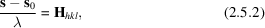where s0 is the unit vector representing the incident beam, s is the unit vector representing the diffracted beam and Hhkl is the reciprocal-lattice vector. Its magnitude is given as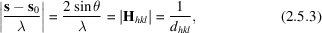in which dhkl is the d-spacing of the crystal planes (hkl). It can be easily seen that it is the Bragg law in a different form. Therefore, equation (2.5.2)is the Bragg law in vector form. In the Bragg condition, the vectors s0/λ and s/λ make angles θ with the diffracting planes (hkl) and Hhkl is normal to the (hkl) crystal plane. In order to analyse all the X-rays measured by a 2D detector, we extend the concept to all scattered X-rays from a sample regardless of the Bragg condition. Therefore, the index (hkl) can be removed from the above expression. H is then a vector which takes the direction bisecting the incident beam and the scattered beam, and has dimensions of inverse length given by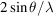. Here 2θ is the scattering angle from the incident beam. The vector H is referred to as the scattering vector or, alternatively, the diffraction vector. When the Bragg condition is satisfied, the diffraction vector is normal to the diffracting lattice planes and its magnitude is reciprocal to the d-spacing of the lattice planes. In this case, the diffraction vector is equivalent to the reciprocal-lattice vector. Each pixel in a 2D detector measures scattered X-rays in a given direction with respect to the incident beam. We can calculate a diffraction vector for any pixel, even if the pixel is not measuring Bragg scattering. Use of the term `diffracted beam' hereafter in this chapter does not necessarily imply that it arises from Bragg scattering.

For two-dimensional diffraction, the incident beam can be expressed by the vector s0/λ, but the diffracted beam is no longer in a single direction, but follows the diffraction cone. Since the direction of a diffraction vector is a bisector of the angle between the incident and diffracted beams corresponding to each diffraction cone, the trace of the diffraction vectors forms a cone. This cone is referred to as the diffraction-vector cone. The angle between the diffraction vector and the incident X-ray beam is 90° + θ and the apex angle of a vector cone is 90° − θ. It is apparent that diffraction-vector cones can only exists on the −XL side of the diffraction space.

For two-dimensional diffraction, the diffraction vector is a function of both the γ and 2θ angles, and is given in laboratory coordinates as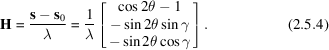The direction of the diffraction vector can be represented by its unit vector, given by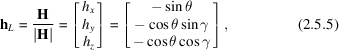where hL is a unit vector expressed in laboratory coordinates and the three components in the square brackets are the projections of the unit vector on the three axes of the laboratory coordinates, respectively. If γ takes all values from 0 to 360° at a given Bragg angle 2θ, the trace of the diffraction vector forms a diffraction-vector cone. Since the possible values of θ lie within the range 0 to 90°, hx takes only negative values.

#### 2.5.2.2. Detector space and pixel position

| top | pdf |

A typical 2D detector has a limited detection surface, and the detection surface can be spherical, cylindrical or flat. Spherical or cylindrical detectors are normally designed for a fixed sample-to-detector distance, while a flat detector has the flexibility to be used at different sample-to-detector distances so as to choose either high resolution at a large distance or large angular coverage at a short distance.

#### 2.5.2.2.1. Detector position in the laboratory system

| top | pdf |

The position of a flat detector is defined by the sample-to-detector distance D and the detector swing angle α. D and α are referred to as the detector-space parameters. D is the perpendicular distance from the goniometer centre to the detection plane and α is a right-handed rotation angle about the ZL axis. Detectors at different positions in the laboratory coordinates XL, YL, ZL are shown in Fig. 2.5.4. The centre of detector 1 is right on the positive side of the XL axis (on-axis), α = 0. Both detectors 2 and 3 are rotated away from the XL axis with negative swing angles (α2 < 0 and α3 < 0). The detection surface of a flat 2D detector can be considered as a plane, which intersects the diffraction cone to form a conic section. Depending on the swing angle α and the 2θ angle, the conic section can appear as a circle, an ellipse, a parabola or a hyperbola.Figure 2.5.4 | top | pdf |Detector positions in the laboratory-system coordinates.

#### 2.5.2.2.2. Pixel position in diffraction space for a flat detector

| top | pdf |

The values of 2θ and γ can be calculated for each pixel in the frame. The calculation is based on the detector-space parameters and the pixel position in the detector. Fig. 2.5.5shows the relationship of a pixel P(x, y) to the laboratory coordinates XL, YL, ZL. The position of a pixel in the detector is defined by the (x, y) coordinates, where the detector centre is defined as x = y = 0. The diffraction-space coordinates (2θ, γ) for a pixel at P(x, y) are given by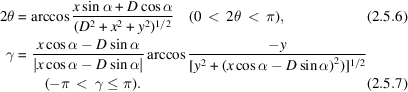Figure 2.5.5 | top | pdf |Relationship between a pixel P and detector position in the laboratory coordinates.

#### 2.5.2.2.3. Pixel position in diffraction space for a curved detector

| top | pdf |

The conic sections of the diffraction cones with a curved detector depend on the shape of the detector. The most common curved detectors are cylinder-shaped detectors. The diffraction frame measured by a cylindrical detector can be displayed as a flat frame, typically a rectangle. Fig. 2.5.6(a) shows a cylindrical detector in the vertical direction and the corresponding laboratory coordinates XL, YL, ZL. The sample is located at the origin of the laboratory coordinates inside the cylinder. The incident X-rays strike the detector at a point O if there is no sample or beam stop to block the direct beam. The radius of the cylinder is R. Fig. 2.5.6(b) illustrates the 2D diffraction image collected with the cylindrical detector. We take the point O as the origin of the pixel position (0, 0). The diffraction-space coordinates (2θ, γ) for a pixel at P(x, y) are given by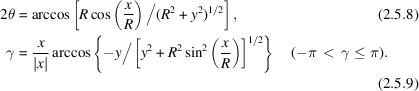The pixel-position-to-(2θ, γ) conversion for detectors of other shapes can also be derived. Once the diffraction-space coordinates (2θ, γ) of each pixel in the curved 2D detector are determined, most data-analysis algorithms developed for flat detectors are applicable to a curved detector as well.Figure 2.5.6 | top | pdf |Cylinder-shaped detector in vertical direction: (a) detector position in the laboratory coordinates; (b) pixel position in the flattened image.

| top | pdf |

#### 2.5.2.3.1. Sample rotations and translations in Eulerian geometry

| top | pdf |

In a 2D-XRD system, three rotation angles are necessary to define the orientation of a sample in the diffractometer. These three rotation angles can be achieved either by a Eulerian geometry, a kappa (κ) geometry or another kind of geometry. The three angles in Eulerian geometry are ω (omega), ψ (psi) and ϕ (phi). Fig. 2.5.7(a) shows the relationship between rotation axes (ω, ψ, ϕ) in the laboratory system XL, YL, ZL. The ω angle is defined as a right-handed rotation about the ZL axis. The ω axis is fixed in the laboratory coordinates. The ψ angle is a right-handed rotation about a horizontal axis. The angle between the ψ axis and the XL axis is given by ω. The ψ axis lies on XL when ω is set at zero. The ϕ angle defines a left-handed rotation about an axis on the sample, typically the normal of a flat sample. The ϕ axis lies on the YL axis when ω = ψ = 0. In an aligned diffraction system, all three rotation axes and the primary X-ray beam cross at the origin of the XL, YL, ZL coordinates. This cross point is also known as the goniometer centre or instrument centre.Figure 2.5.7 | top | pdf |Sample rotation and translation. (a) Three rotation axes in laboratory coordinates; (b) rotation axes (ω, ψ, ϕ) and sample coordinates.

Fig. 2.5.7(b) shows the relationship and stacking sequence among all rotation axes (ω, ψ, ϕ) and the sample coordinates S1, S2, S3. ω is the base rotation; all other rotations and translations are on top of this rotation. The next rotation above ω is the ψ rotation. The next rotation above ω and ψ is the ϕ rotation. The sample coordinates S1, S2, S3 are fixed to the sample regardless of the particular sample orientation given by the rotation angles (ω, ψ, ϕ). The ϕ rotation in the goniometer is intentionally chosen as a left-handed rotation so that the diffraction vectors will make a right-hand rotation observed in the sample coordinates S1, S2, S3.

| top | pdf |

#### 2.5.2.4.1. Diffraction unit vector in diffraction space and sample space

| top | pdf |

In 2D-XRD data analysis, it is crucial to know the diffraction-vector distribution in terms of the sample coordinates S1, S2, S3. However, the diffraction-vector distribution corresponding to the measured 2D data is always given in terms of the laboratory coordinates XL, YL, ZL because the diffraction space is fixed to the laboratory coordinates. Fig. 2.5.8shows the unit vector of a diffraction vector in both (a) the laboratory coordinates XL, YL, ZL and (b) the sample coordinates S1, S2, S3. In Fig. 2.5.8(a) the unit vector hL is projected to the XL, YL and ZL axes as hx, hy and hz, respectively. The three components are given by equation (2.5.5). In order to analyse the diffraction results relative to the sample orientation, it is necessary to transform the unit vector to the sample coordinates S1, S2, S3. Fig. 2.5.8(b) shows the same unit vector, denoted by hs projected to S1, S2 and S3 as h1, h2 and h3, respectively.Figure 2.5.8 | top | pdf |Unit diffraction vector in (a) the laboratory coordinates and (b) the sample coordinates.

#### 2.5.2.4.2. Transformation from diffraction space to sample space

| top | pdf |

The transformation of the unit diffraction vector from the laboratory coordinates XL, YL, ZL to the sample coordinates S1, S2, S3 is given by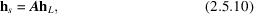where A is the transformation matrix. For Eulerian geometry in matrix form, we have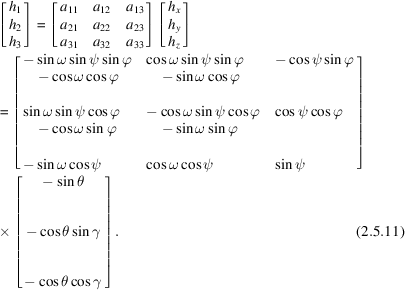In expanded form: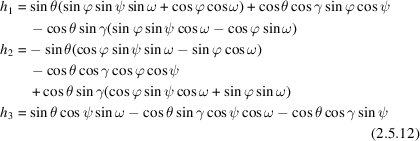In addition to the diffraction intensity and Bragg angle corresponding to each data point on the diffraction ring, the unit vector hs{h1, h2, h3} provides orientation information in the sample space. The transformation matrix of any other goniometer geometry, such as kappa geometry (Paciorek et al., 1999), can be introduced into equation (2.5.10)so that the unit vector hs{h1, h2, h3} can be expressed in terms of the specified geometry. All equations using the unit vector hs{h1, h2, h3} in this chapter, such as in data treatment, texture analysis and stress measurement, are applicable to all goniometer geometries provided that the unit-vector components are generated from the corresponding transformation matrix from diffraction space to the sample space.

#### 2.5.2.4.3. Transformation from detector space to reciprocal space

| top | pdf |

Reciprocal-space mapping is commonly used to analyse the diffraction patterns from highly oriented structures, diffuse scattering from crystal defects, and thin films (Hanna & Windle, 1995; Mudie et al., 2004; Smilgies & Blasini, 2007; Schmidbauer et al., 2008). The equations of the unit-vector calculation given above can also be used to transform the diffraction intensity from the diffraction space to the reciprocal space with respect to the sample coordinates. The direction of the scattering vector is given by the unit vector hs{h1, h2, h3} and the magnitude of the scattering vector is given by, so that the scattering vector corresponding to a pixel is given by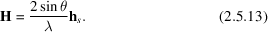The three-dimensional reciprocal-space mapping can be obtained by applying the normalized pixel intensities to the corresponding reciprocal points. With various sample orientations, all pixels on the detector can be mapped into a 3D reciprocal space.

### References

Hanna, S. & Windle, A. H. (1995). A novel polymer fibre diffractometer, based on a scanning X-ray-sensitive charge-coupled device. J. Appl. Cryst. 28, 673–689.Google Scholar
He, B. B. (2003). Introduction to two-dimensional X-ray diffraction. Powder Diffr. 18, 71–85.Google Scholar
Mudie, S. T., Pavlov, K. M., Morgan, M. J., Hester, J. R., Tabuchi, M. & Takeda, Y. (2004). Collection of reciprocal space maps using imaging plates at the Australian National Beamline Facility at the Photon Factory. J. Synchrotron Rad. 11, 406–413.Google Scholar
Paciorek, W. A., Meyer, M. & Chapuis, G. (1999). On the geometry of a modern imaging diffractometer. Acta Cryst. A55, 543–557.Google Scholar
Schmidbauer, M., Schäfer, P., Besedin, S., Grigoriev, D., Köhler, R. & Hanke, M. (2008). A novel multi-detection technique for three-dimensional reciprocal-space mapping in grazing-incidence X-ray diffraction. J. Synchrotron Rad. 15, 549–557.Google Scholar
Smilgies, D.-M. & Blasini, D. R. (2007). Indexation scheme for oriented molecular thin films studied with grazing-incidence reciprocal-space mapping. J. Appl. Cryst. 40, 716–718.Google Scholar